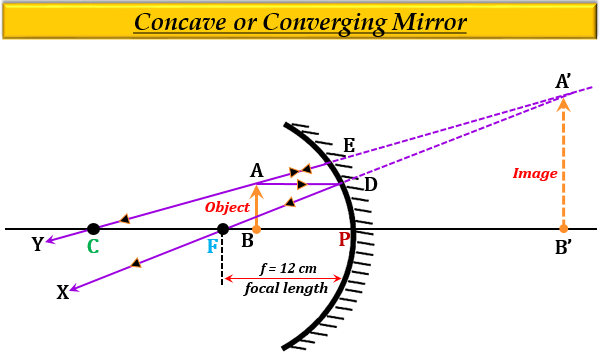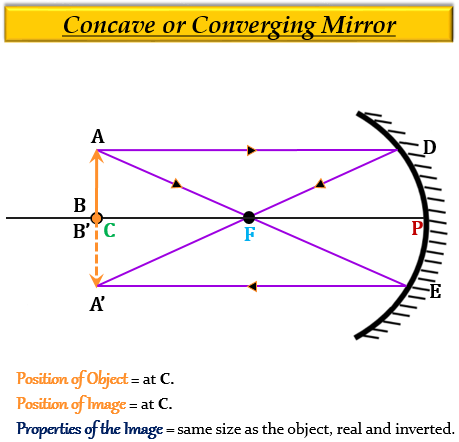# It is desired to obtain an erect image of an object, using concave mirror of focal length of 12 cm.(i) What should be the range of distance of an object placed in front of the mirror?(ii) Will the image be smaller or larger than the object? Draw ray diagram to show the formation of image in this case.(iii) Where will the image of this object be, if it is placed 24 cm in front of the mirror? Draw ray diagram for this situation also justify your answer.Show the positions of pole, principal focus, and the centre of curvature in the above ray diagrams.

(i) To obtain an erect image of an object, using concave mirror of focal length of 12 cm, the range of the object distance placed in front of the mirror is from 0 to 12 cm. This is because in concave mirror erect image is formed only when the object is placed between the pole $(P)$ and the focus $(F)$. Therefore, object distance should be less than 12 cm.

(ii) The nature of the image formed will be magnified (larger than the size of the object).(iii) If the object is placed 24 cm in front of the mirror which is the approx position of centre of curvature $(C,\ or\ 2f)$, then the image will be formed at the same position, that is at center of curvature of the mirror.Updated on: 10-Oct-2022

7K+ Views